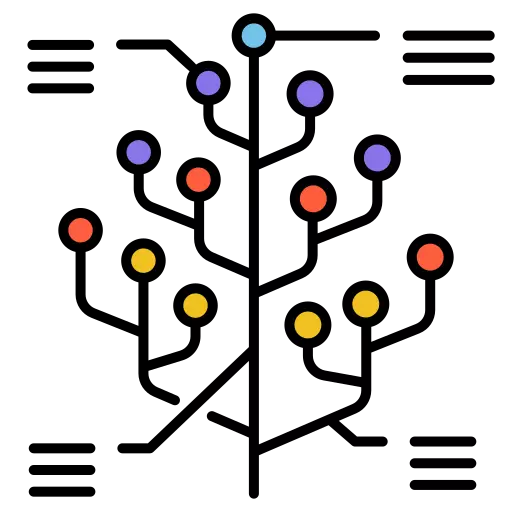# Lowest Common Ancestor in a Binary Tree

## What is Lowest Common Ancestor (LCA):

The Lowest Common Ancestor (LCA) in a binary tree is the lowest (deepest) node in the tree that has both of the given nodes as descendants. In other words, it’s the node that is a common ancestor of the two nodes and is the farthest from the root. The LCA is often used to determine the relationship between two nodes in a binary tree.

In this article, you will learn about the Lowest Common Ancestor in a Binary Tree and its example and Approach of the Problems.### Understanding Lowest Common Ancestor in deep:

Imagine you have a college’s academic hierarchy, which can be represented as a binary tree. Each person in the hierarchy, such as students and professors, is a node in this binary tree. You want to find the “lowest common ancestor” (LCA) of two students in the college.

The lowest common ancestor (LCA) in a binary tree is a concept used to find the shared ancestor of two nodes in the tree that is the closest to both of them. In simpler terms, it’s like finding the “meeting point” or “parent” of two nodes in a family tree.

## Example of Lowest Common Ancestor:### In this hierarchy:

• The LCA of students X and Y is “Prof A” because it’s the earliest professor they both share in common. This professor is at the lowest academic level, closest to students X and Y.
• So, in this scenario, the LCA helps determine the academic relationship between two students, showing which professor is their closest shared advisor or mentor.

### Applications of Lowest Common Ancestor:

The Lowest Common Ancestor (LCA) in a binary tree is a fundamental concept used in various applications and algorithms in computer science and data structures. Here are some of the key applications of LCA:

1. Binary Tree Traversal: LCA can be used to optimize binary tree traversal algorithms. For example, if you need to find the distance between two nodes in a binary tree, you can first find their LCA and then calculate the distances from the LCA to both nodes. This reduces the overall time complexity.

2. Binary Tree Construction: When constructing binary trees from their various representations, such as preorder and inorder traversals or postorder and inorder traversals, LCA can help determine the root of the tree efficiently.

3. Lowest Common Ancestor Queries: LCA is used in various algorithms to answer queries about the relationships between nodes in a binary tree. For example, determining the LCA of two nodes can help find the nearest common ancestor in genealogy or the most recent common ancestor in evolutionary biology.

4. Graph Algorithms: LCA can be extended to solve problems in graph theory. For instance, finding the LCA of two nodes in a directed acyclic graph (DAG) can help in determining the lowest common ancestor in a directed graph, which is useful in various applications like dependency resolution and dynamic programming.

##### Testcase 1
```Input:
3
/ \
5   1
/ \ / \
6  2 0  8
/\
7  4
Nodes: 5 (nodeA) and 1 (nodeB)
Expected Output: The LCA is 3```

### Approach:

Input: You are given a binary tree represented by a data structure with nodes. You also have two nodes, nodeA and nodeB, for which you want to find the LCA.

1. Start at the root of the binary tree.
2. Recursively search for both target nodes in the left and right subtrees.
3. The first common ancestor you encounter while searching is the Lowest Common Ancestor (LCA).

This approach efficiently finds the LCA in a binary tree by searching for the target nodes and identifying their common ancestor.

### Related Banners

Get PrepInsta Prime & get Access to all 200+ courses offered by PrepInsta in One Subscription

Run
```class TreeNode:
def __init__(self, val):
self.val = val
self.left = None
self.right = None

def findLowestCommonAncestor(root, p, q):
if root is None:
return None

if root.val == p.val or root.val == q.val:
return root

left_lca = findLowestCommonAncestor(root.left, p, q)
right_lca = findLowestCommonAncestor(root.right, p, q)

if left_lca and right_lca:
return root

return left_lca if left_lca else right_lca

# Test Cases
# Create a binary tree
root = TreeNode(3)
root.left = TreeNode(5)
root.right = TreeNode(1)
root.left.left = TreeNode(6)
root.left.right = TreeNode(2)
root.right.left = TreeNode(0)
root.right.right = TreeNode(8)
root.left.right.left = TreeNode(7)
root.left.right.right = TreeNode(4)

# Test Case 1: LCA of nodes 5 and 1
nodeA = root.left
nodeB = root.right
lca = findLowestCommonAncestor(root, nodeA, nodeB)
print("Test Case 1:", lca.val)  # Expected output: 3

# Test Case 2: LCA of nodes 5 and 4
nodeA = root.left
nodeB = root.left.right.right
lca = findLowestCommonAncestor(root, nodeA, nodeB)
print("Test Case 2:", lca.val)  # Expected output: 5

# Test Case 3: LCA of nodes 2 and 8
nodeA = root.left.right
nodeB = root.right.right
lca = findLowestCommonAncestor(root, nodeA, nodeB)
print("Test Case 3:", lca.val)  # Expected output: 3

# Test Case 4: LCA of nodes 7 and 0
nodeA = root.left.right.left
nodeB = root.right.left
lca = findLowestCommonAncestor(root, nodeA, nodeB)
print("Test Case 4:", lca.val)  # Expected output: 3
```

## Get over 200+ course One Subscription

Courses like AI/ML, Cloud Computing, Ethical Hacking, C, C++, Java, Python, DSA (All Languages), Competitive Coding (All Languages), TCS, Infosys, Wipro, Amazon, DBMS, SQL and others